Study Guide

# Polynomials - Exponents

## Exponents

If you're still not sure what exponents are, feel free to travel back in time to our handy exponent guide. We're traveling to whatever planet polynomials are from in this little fantasy of ours, so we don't see why you shouldn't be able to time travel as well. Bring back some baseball stats for us!

In addition to the whole number exponents we talked about earlier, exponents can also be negative, rational, and even irrational.

While they can get freaky, exponents still play by some rules.

Rule #1: The Additive Exponent Rule. Multiplying two powers of a number is the same as adding the exponents.

In symbols, this says that (xa)(xb) = xa + b. Here's a real number example:

(75)(73) = 75 + 3 = 78

Rule #2: The Multiplicative Exponent Rule. If you're taking the power of an exponent (the exponent of something that's already raised to an exponent), then you multiply those exponents together.

In symbols, this is (xa)b = xab. Or, in real numbers, (142)50 = 14100.

• ### Negative Exponents

Negative exponents are basically abbreviations for big ugly fractions. In the same way that it's quicker to write LMAO in place of the longer version, negative exponents will make it much easier to text all your friends about algebra. We know that's what 95% of all your texts are about. You're so obsessed.

Let's start with a simple example that showcases the additive rule: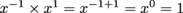In other words,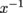is the multiplicative inverse or reciprocal of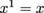. Therefore,. Got that, Fraction Jackson?

### Sample Problems

•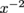, that is,, is the reciprocal of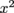.

•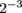means.

•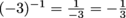Make sure you put negative exponents in that part of your brain where you keep things, right in between the cheat codes for Street Fighter and the fantasy stats for every professional baseball player. They'll be important when you learn scientific notation, which we'll talk about later. You'll need to hang in there and notate stuff unscientifically until then.

• ### Fractional Exponents

Fractional exponents are abbreviations for taking the roots of a number. For example, x½ means, the square root of x. This makes sense because, by the rules of addition for exponents:

(x½)(x½) = x(½ + ½) = x1 = x

In the same way, x is the cube root of x. We need to multiply x by itself three times to get back to x. Moving along, x¼ is the fourth root of x, x is the fifth root of x, and so on. What's that? You want one more? All right, all right: x is the sixth root of x, but that's all you're getting from us.

What about fractions that have a number other than 1 in the numerator? Can we ignore them and move on to easier problems? Unfortunately, no. That big gaping hole on your answer sheet will be glaring. What would x¾ mean? Well, if we multiply x¾ by itself 4 times, we get x3. Therefore, x¾ is still a root; it's the fourth root of x cubed. By the rules of multiplying exponents, we can also write x¾ as (x3)¼, or as (x¼)3.

We could write it many other ways as well, but those other ways would be wrong, and if simplifying an exponent is wrong, we don't want to be right. Wait, what?

### Sample Problems

The expression 8 can also be written as  (8)2 . Since the cube root of 8 is 2, this simplifies to (2)2 = 4.

The expressioncan be written aswhich simplifies to.

• ### Irrational Exponents

Let's kick things up a notch. It's time to introduce irrational exponents. You'll never need another Red Bull again if you can get addicted to these bad boys. What does 2π mean, for example? We can't possibly multiply π copies of 2 together, can we? Even if we could, wouldn't that get awfully messy?

While we can't write out all the digits of π, we can approximate π using decimals:

π ≈ 3 (not a great approximation)
π ≈ 3.1
π ≈ 3.14
π ≈ 3.141 and so on.

We can use these approximations of π to approximate 2π.

2π ≈ 23
2π ≈ 23.1
2π ≈ 23.14
2π ≈ 23.141

Since our approximations of π are rational numbers, we can find 23, 23.1, and so on. We'll never be able to write down all the digits of 2π, in the same way that we'll never be able to write down all the digits of π. Don't beat yourself up about it. Do what mathematicians do. Throw your hands in the air, give up on the thought of arriving at an exact answer, and PART-AY!

Uh, estimate. That last one was supposed to be "estimate."

• ### Variables as Exponents

Since exponents can be any real number and variables are basically the alien decoys of real numbers, we can write down expressions like 2x. We don't love to do it, but we can. We can evaluate these expressions for given values of x, multiply them together, and do whatever else we want to do with them as long as our mother approves. Parents first, algebra second.

### Sample Problem

What is (3x)(34x)?

Since the base is the same, we add the exponents to get 35x. Two players with the same base...that would never fly in baseball. Fortunately, in algebra, they can pile onto the same base as much as they like.